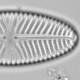## Keenan’s test with added drift

Doug Keenan visited to defend his methods. His main arguments were argument from vicarious authority and argument by condescension, but he also left this:

“The ARIMA model is specified to be driftless: this means that the drift (i.e. average of the first differences of the series) in the model is 0. Your claim that differencing the series removes a trend makes no sense: if there was a trend, the drift would obviously not be 0.”

The mean of the first differences is 0.005 °C/yr. Obviously not zero, but Keenan argues that “inferences are not drawn directly from the data. Rather, a statistical model is fit to the data and then inferences are drawn from the model.” Fair enough, so I’m going to add a linear trend to the HadCrut4 data to determine how much extra linear trend is required before the linear trend model has a lower AIC than the ARIMA(3,1,0) model.

```res<-sapply(0:50, function(n){
mod2<-arima(y, order=c(3,1,0))
c(AIC(mod1), AIC(mod2))
})
x11(4,4);par(mar=c(3,3,1,1), mgp=c(1.5,.5,0))
matplot(0:50, t(res), type="l", lty=1, col=c(4,2), ylab="AIC", xlab="Added temperature change 1850-2013")
legend("bottomright", legend=c("Linear trend", "ARIMA"), lty=1, col=c(4,2), bty="n")
```However much linear trend is added to the data the AIC of the ARIMA(3,1,0) model is always below that of the linear model. Even when we know that drift is non-zero because we added huge amount of it, Keenan’s test would still show that the ARIMA(3,1,0) model was better than the linear model. This is absurd. Keenan’s test has no power. Either it is hopelessly sensitive to non-linearities in the data, or as Gavin suggested, the AIC of the linear model and the ARIMA model are not directly compatible. Whatever the explanation is, the test is not fit for purpose.HadCrut4 data with an extra 50°C increase. Still not significant according to Keenan’s methods.Ecologist with interests in quantitative methods and palaeoenvironments
This entry was posted in climate, Fake climate sceptics, R, Silliness and tagged , . Bookmark the permalink.

### 11 Responses to Keenan’s test with added drift

1.wottsupwiththatblog says:

Silly question, but what is AIC? Also, is there is simple way to explain the ARIMA(3,1,0) model/method as I’m really not getting what Keenan is doing with this particular analysis (although maybe that is essentially the point you’re trying to make).

•richard telford says:

AIC (Akaike information criterion) is a measure of how well the model fits the data, with a penalty for model complexity. It can be used for model selection: models with a lower AIC being preferred.
Keenan’s model first finds the difference in the temperature between each year and the previous year and then fits an autoregressive model to these differences. It is the first step that is most important as it will very effectively remove any trend in the data, as I showed in the first figure of this post.

•wottsupwiththatblog says:

Thanks. So I’ve briefly reread Keenan’s WSJ oped piece and he does seem to say “what if we analyse the changes rather than the temperatures themselves”. I presume that by “change” he means the difference between one data point and the next, rather than the anomaly itself (which is what I think he means by the term temperature). Simplistically, I can see how this would remove a trend as the differences in a pure straight line would be constant, which I assume he would interpret as, therefore, trendless. Is this essentially what he has done?

•richard telford says:

yes

•wottsupwiththatblog says:

Well that would seem to explain it then 🙂

2.Nick Stokes says:

Richard,
I did a test here in which I extended Keenan’s treatment with drift, and tested the drift for significant deviation from zero, much as one would test a trend. The value was close to the OLS trend, and the result fell just below significance, on a naive test. But in fact there is a 4×4 covariance matrix, and the diagonal is dominant, but not totally. So I think it just means that a (3,1,0) model is better able to emulate a trend than a (1,0,0) model would be.

•richard telford says:

The model with the lowest AIC I have found is an ARMA(3,1) model with drift:
 -271.8758
 -273.2807
No need for differencing! I suspect that using the forcing data rather than a linear trend would improve the fit and need a simpler ARMA model.

•ucfagls says:

…but as those models are essentially within 2 AIC units (where comparable likelihood calculation was used) they are essentially doing as well at fitting the data. In this case, the first-order differencing and the drift are accounting for the same linear trend component in the data. IIRC, the mean of the differenced series should be the slope of the linear trend in the drift model – the differencing renders this trend constant in the resultant series, but that series is now offset from 0 by that value of the slope.

This is why I just don’t understand what Keenan is trying to say/do with this analysis. The bit he is harping on about, the ARMA(3,0) is for a stochastic process after the (linear) trend in the data is removed. However, it is the trend in the data that we are interested in.

To be somewhat flippant; the only useful thing Keenan mentions in his piece is that a linear model with AR(1) residuals is not a good fit to these data. I think we can all agree on that!?

•James says:

ucfagls, yes. That is Keenan’s main point, AR(1) is not a good model, but that’s what the IPCC and Met Office use.

•ucfagls says:

Even that has been contested… And that wasn’t his point, otherwise he’d have stopped at showing that linear model + AR(1) residuals is not a good fit. He tried to make another point, involving ARIMA(3,1,0) and other things. A locally linear (or spline) model with AR(1) residuals does fit the data well and does account for the residual correlation.